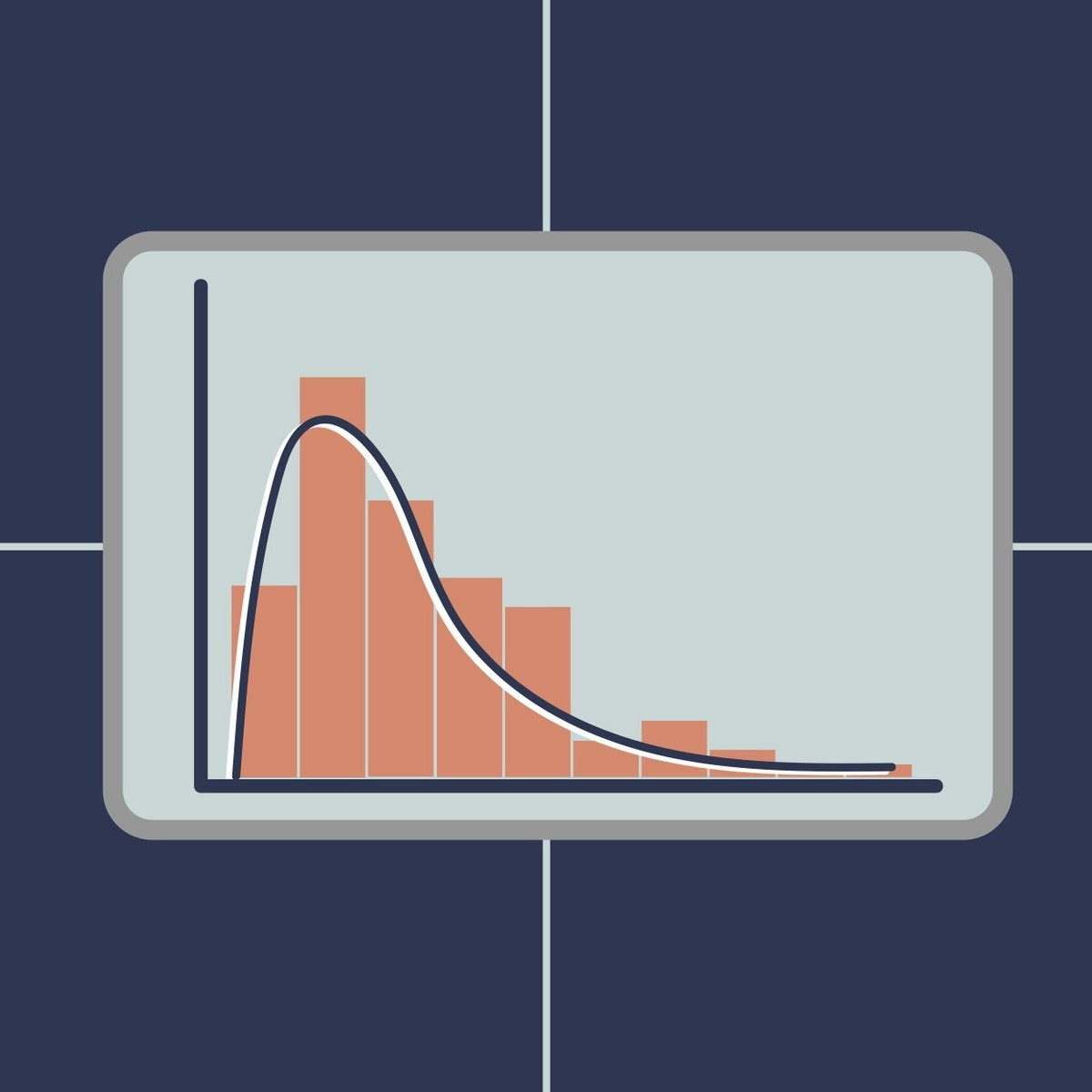# Bayesian Statistics: Techniques and Models

University of California, Santa Cruz

This is the second of a two-course sequence introducing the fundamentals of Bayesian statistics. It builds on the course Bayesian Statistics: From Concept to Data Analysis, which introduces Bayesian methods through use of simple conjugate models. Real-world data often require more sophisticated models to reach realistic conclusions. This course aims to expand our “Bayesian toolbox” with more general models, and computational techniques to fit them. In particular, we will introduce Markov chain Monte Carlo (MCMC) methods, which allow sampling from posterior distributions that have no analytical solution. We will use the open-source, freely available software R (some experience is assumed, e.g., completing the previous course in R) and JAGS (no experience required). We will learn how to construct, fit, assess, and compare Bayesian statistical models to answer scientific questions involving continuous, binary, and count data. This course combines lecture videos, computer demonstrations, readings, exercises, and discussion boards to create an active learning experience. The lectures provide some of the basic mathematical development, explanations of the statistical modeling process, and a few basic modeling techniques commonly used by statisticians. Computer demonstrations provide concrete, practical walkthroughs. Completion of this course will give you access to a wide range of Bayesian analytical tools, customizable to your data.

Certificate Available ✔#### Introduction to Computational Statistics for Data Scientists

Databricks

The purpose of this series of courses is to teach the basics of Computational Statistics for the purpose of performing inference to aspiring or new Data Scientists....

Eindhoven University of Technology

This course aims to help you to draw better statistical inferences from empirical research. First, we will discuss how to correctly interpret p-values, effect sizes,...